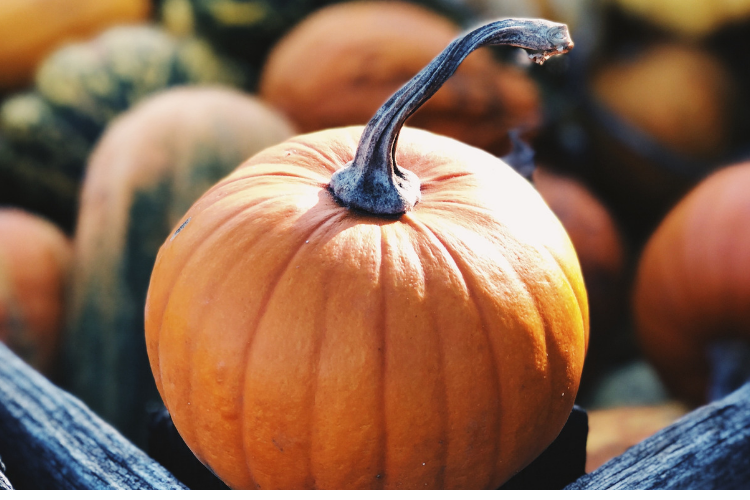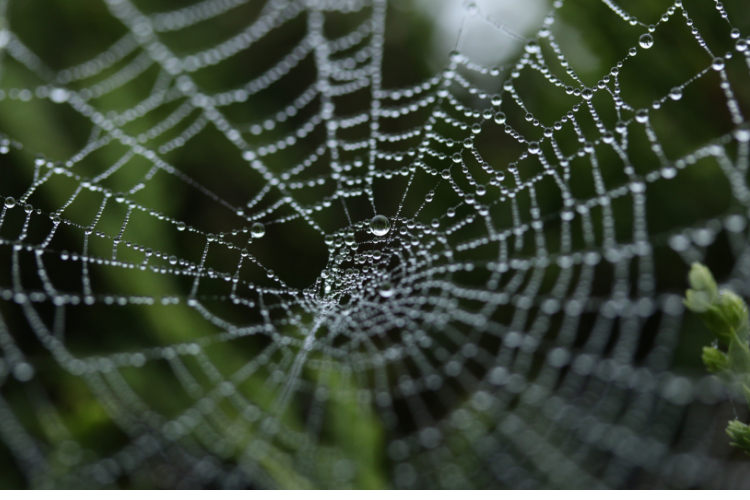It can be hard to get kids excited about math. Spice up the lesson with Halloween vibes!

To take traditional math lessons and turn it festive use pumpkins, spiders, and spider webs.### PUMPKIN GAMES

With one pumpkin, you can employ the following 3 games.

How much does it weigh?

Take turns holding a pumpkin and guessing how much it weighs. Then, find the actual weight by using a scale.

See who had the closest estimate as well as the difference between the actual weight and closest prediction.

How big is my waistline?

Estimate the circumference of a pumpkin at its widest point. Then, use a tape measure to get the actual circumference.

How many seeds?

Divide the class into groups. Cut open a pumpkin and ask each group to estimate how many seeds are inside. Then give each group a portion of the seeds to count.

After adding up the group totals, find out who had the closest estimate of the total of seeds and how far it was from the actual number.### SPIDER MATH

Get crafty using graph papers and spiders.

Symmetrical spiders

Have each student draw perfectly symmetrical spiders using graph paper. Make sure that each line segment begins and ends at an intersection of the graph.

Then, trade spiders with a partner and see if everyone can copy their partner’s spider with each point at the right coordinate of the graph paper.

Making spider webs

Before class, find interesting math facts about a spider’s spinners and the thickness, strength, elasticity, and quantity of its silk. You can make a poster or fact sheet on the math of making a spider web and examples of different kinds of spider webs.

In class, have your students draw a web of their choice on graph paper.

via education world